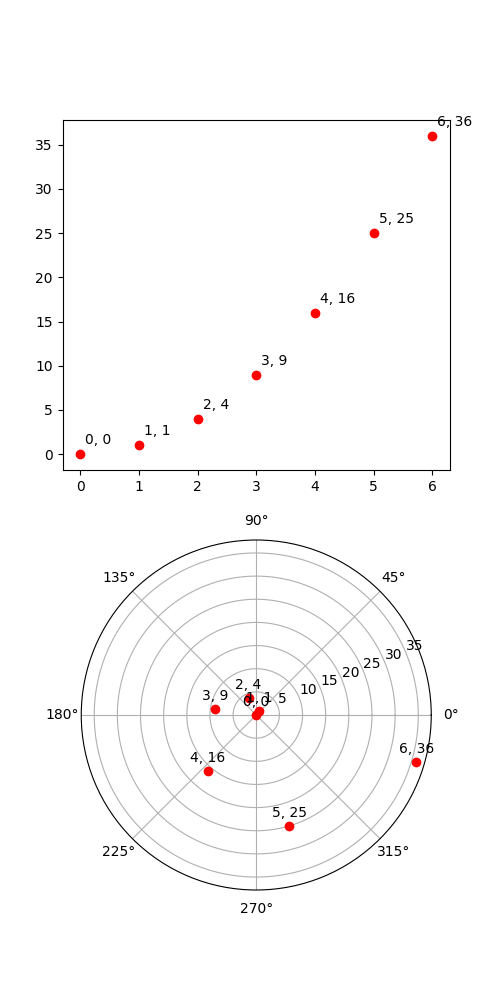Transoffset¶

This illustrates the use of transforms.offset_copy to make a transform that positions a drawing element such as a text string at a specified offset in screen coordinates (dots or inches) relative to a location given in any coordinates.

Every Artist–the mpl class from which classes such as Text and Line are derived–has a transform that can be set when the Artist is created, such as by the corresponding pyplot command. By default this is usually the Axes.transData transform, going from data units to screen dots. We can use the offset_copy function to make a modified copy of this transform, where the modification consists of an offset.import matplotlib.pyplot as plt
import matplotlib.transforms as mtransforms
import numpy as np

from matplotlib.transforms import offset_copy

xs = np.arange(7)
ys = xs**2

fig = plt.figure(figsize=(5, 10))
ax = plt.subplot(2, 1, 1)

# If we want the same offset for each text instance,
# we only need to make one transform.  To get the
# transform argument to offset_copy, we need to make the axes
# first; the subplot command above is one way to do this.
trans_offset = mtransforms.offset_copy(ax.transData, fig=fig,
x=0.05, y=0.10, units='inches')

for x, y in zip(xs, ys):
plt.plot((x,), (y,), 'ro')
plt.text(x, y, '%d, %d' % (int(x), int(y)), transform=trans_offset)

# offset_copy works for polar plots also.
ax = plt.subplot(2, 1, 2, projection='polar')

trans_offset = mtransforms.offset_copy(ax.transData, fig=fig,
y=6, units='dots')

for x, y in zip(xs, ys):
plt.polar((x,), (y,), 'ro')
plt.text(x, y, '%d, %d' % (int(x), int(y)),
transform=trans_offset,
horizontalalignment='center',
verticalalignment='bottom')

plt.show()

Keywords: matplotlib code example, codex, python plot, pyplot Gallery generated by Sphinx-Gallery